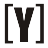# Kilograms (kg) to Tons (t) Conversion

How to Convert Kilograms to Tons (Metric) [t]: insert number in Kilograms box and press convert to conversion into Tons (t).

 Kilograms: kg Tonnes: t Calculation:

## How to convert kg to Tons

1 kg = 0.001 Tons (t)
1 t = 1000 kg

Formula
m (t) = m (kg) / 1000

The mass m in kilograms (kg) divided by 1000

Example: Convert 1500 kg to tons?
1500 kg = 1500 kg / 1000 = 1.5 t
1500 kg is 1.5 tons.

kg to pounds conversion table

 Kilograms (kg) 0 kg 0.1 kg 1 kg 2 kg 3 kg 4 kg 5 kg 6 kg 7 kg 8 kg 9 kg 10 kg 20 kg 30 kg 40 kg 50 kg 60 kg 70 kg 80 kg 90 kg 100 kg 1000 kg Tons (t) 0 t 0.0001 t 0.001 t 0.002 t 0.003 t 0.004 t 0.005 t 0.006 t 0.007 t 0.008 t 0.009 t 0.01 t 0.02 t 0.03 t 0.04 t 0.05 t 0.06 t 0.07 t 0.08 t 0.09 t 0.1 t 1 tYoosFuhl.com Would you like to receive notifications on latest updates? No Yes